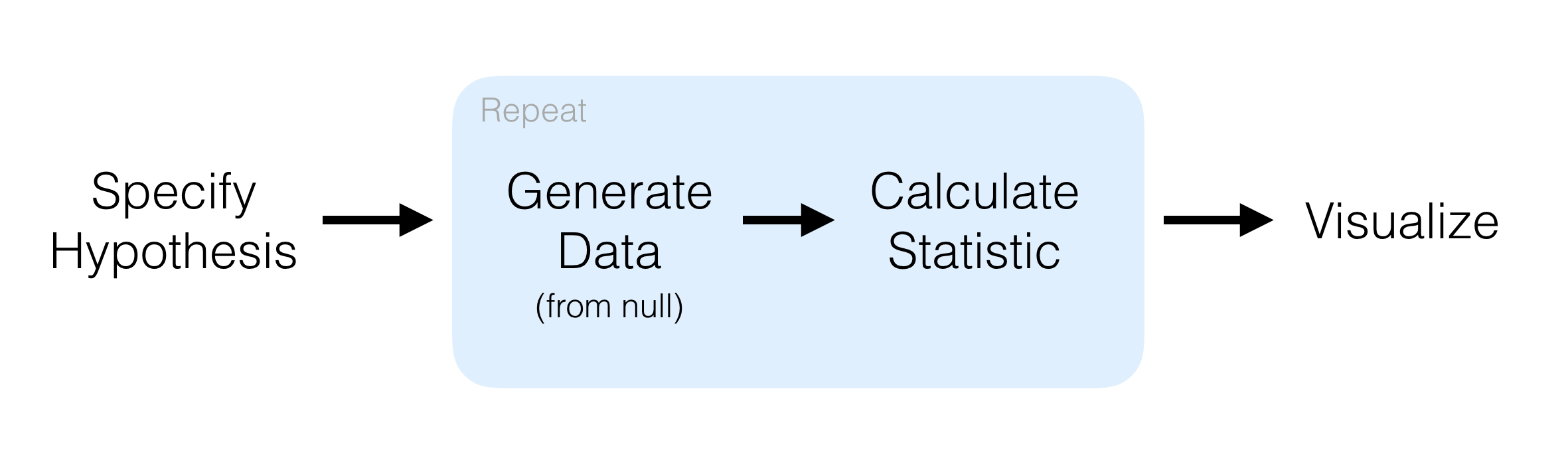The objective of this package is to perform statistical inference using an expressive statistical grammar that coheres with the tidyverse design framework.### Installation

To install the current stable version of infer from CRAN:

install.packages("infer")

To install the developmental version of infer, make sure to install remotes first. The pkgdown website for this developmental version is at https://infer.netlify.com.

install.packages("remotes")
remotes::install_github("tidymodels/infer")

To install the cutting edge version of infer (do so at your own risk), make sure to install remotes first. This version was last updated on 2019-03-12 20:28:54.

install.packages("remotes")
remotes::install_github("tidymodels/infer", ref = "develop")

To see the things we are working on with the package as vignettes/Articles, check out the developmental pkgdown site at https://infer-dev.netlify.com.

### Contributing

We welcome others helping us make this package as user friendly and efficient as possible. Please review our contributing and conduct guidelines. Of particular interest is helping us to write testthat tests and in building vignettes that show how to (and how NOT to) use the package. By participating in this project you agree to abide by its terms.

### Examples

These examples assume that mtcars has been overwritten so that the variables cyl, vs, am, gear, and carb are factors.

mtcars <- as.data.frame(mtcars) %>%
mutate(cyl = factor(cyl),
vs = factor(vs),
am = factor(am),
gear = factor(gear),
carb = factor(carb))

Hypothesis test for a difference in proportions (using the formula interface y ~ x in specify()):

mtcars %>%
specify(am ~ vs, success = "1") %>%
hypothesize(null = "independence") %>%
generate(reps = 100, type = "permute") %>%
calculate(stat = "diff in props", order = c("1", "0"))

Confidence interval for a difference in means (using the non-formula interface giving both the response and explanatory variables in specify()):

mtcars %>%
specify(response = mpg, explanatory = am) %>%
generate(reps = 100, type = "bootstrap") %>%
calculate(stat = "diff in means", order = c("1", "0"))

Note that the formula and non-formula interfaces work for all implemented inference procedures in infer. Use whatever is more natural for you. If you will be doing modeling using functions like lm() and glm(), we recommend you begin to use the formula y ~ x notation as soon as possible though.

Other examples are available in the package vignettes.